# Benchmark: Process Costs for Searching Values in Spreadsheet using Google Apps Script

Gists

## Introduction

Here, I would like to report the process costs for searching values in Spreadsheet using Google Apps Script (GAS). When the values are searched in Google Spreadsheet, the following 3 patterns can be considered. Ref

1. Retrieve all values using getValues, and the values are searched from the retrieved array.
2. Use TextFinder.
3. Use Query language.

In these cases, it has already been found that the lowest process cost is to use the Query language. And about finding values from an array, I have already been reported as “Benchmark: Search for Array Processing using Google Apps Script”. But I had never summarized the process costs for TextFinder and find values from an array. So in this report, I would like to introduce this. As the result, the importance of TextFinder for retrieving the row numbers and the row values by searching a value could be understand.

## Experimental procedure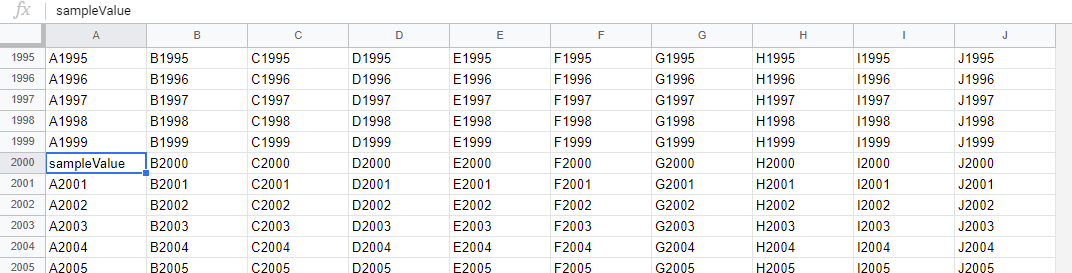4 sample Spreadsheets of the 10,000, 40,000, 70,000 and 100,000 rows and 10 columns were used for measuring the process cost. In each Spreadsheet, the search value is put every 2000 rows, and the search value is set to the column “A”, “E” and “J”. For example, in the case of the sample Spreadsheet of 10,000 rows, the search value is set to the columns “A”, “E” and “J” for 2000, 4000 and 6000 rows, respectively. The process costs to search the search value from these Spreadsheet are measured.

In this experiment, the sample scripts for GAS were used with enabling V8 runtime. The scripts for measuring the search costs of TextFinder and finding values from an array can be seen at Appendix section. Ref

By the way, at GAS, the processing time is not stable as you know. So the average value for more than 200 times measurements was used for each data point which is shown by figures. At this time, the fluctuation of the average values was less than 1 %. I worry that each detailed-data point at my environment might be different from that at other user’s environment. But I think that the trend of this result can be used.

## Results and discussions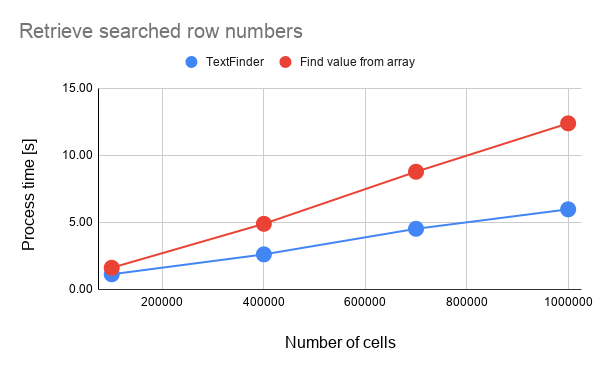Fig 1. Process costs for retrieving searched row numbers. Blue and red lines are for TextFinder (script) and find values from array (script), respectively.

From Fig. 1, it is found that when the row numbers are retrieved by searching values, the process cost of TextFinder is lower than that of find values from array. In this case, the cost can be reduced about 55 % for the cost of “find value from array” in the average.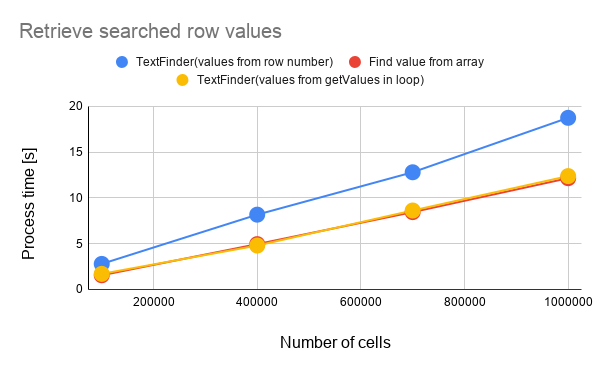Fig 2. Process costs for retrieving searched row values. Blue, yellow and red lines are for TextFinder (values from row number. script), TextFinder (values from getValues in loop. script) and find values from array (script), respectively.

From Fig. 2, it is found that when the row values are retrieved by searching values, the process cost is different from that of Fig. 1. The cost of TextFinder (values from row number) is higher than that of “find value from array”. In the case of TextFinder (values from row number), the row values are retrieved from const values = sheet.getDataRange().getValues(). On the other hand, in the case of TextFinder(values from getValues in loop), the cost is lower than that of “values from row number”. This means that the cost of sheet.getRange(range.getRow(), 1, 1, col).getValues() in the loop is lower than that of method for retrieving the row values using the index of array. This is the interesting result. This result indicates that the size of range strongly affects to the process cost. Because when the range of the same size is retrieved, the cost of getDataRange are almost the same with that of getRange.

As the next step, the process costs of the method for retrieving row numbers and row values are compared. Figure 3 shows the process costs for retrieving searched row numbers and row values in TextFinder.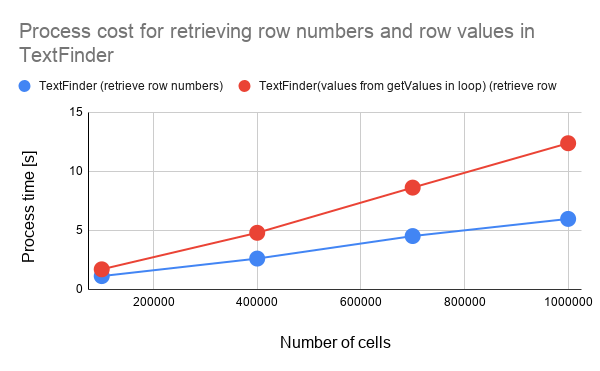Fig 3. Process costs for retrieving searched row numbers and row values in TextFinder. Blue and red lines are for the cost for retrieving row numbers script and row values script, respectively.

When the Fig. 3 is seen, it is found that the cost for retrieving the row numbers is lower than that for retrieving the row values.

Figure 4 shows the process costs for retrieving searched row numbers and row values in “find value from array”.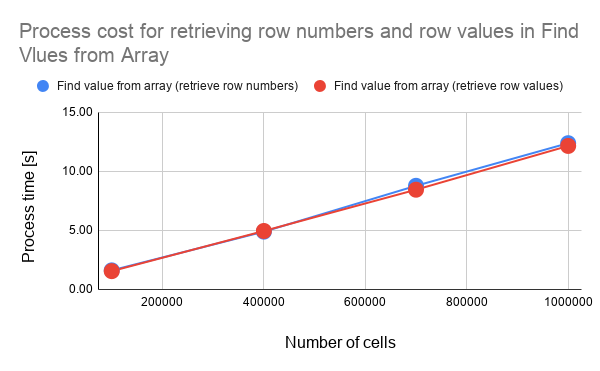Fig 4. Process costs for retrieving searched row numbers and row values in “find value from array”. Blue and red lines are for the cost for retrieving row numbers script and row values script, respectively.

When the Fig. 4 is seen, it is found that the cost for retrieving the row numbers is almost the same with that for retrieving the row values. In this case, the difference is that the value or the index are retrieved from the values retrieved with .getDataRange().getValues(). By this, the cost becomes almost the same. But at the TextFinder, only range list is returned from .createTextFinder(findText).findAll(). By this, the cost for retrieving the values from the range list is increased for the method for retrieving the row numbers.

## Summary

In this report, I investigated the process costs of search value from Spreadsheet using TextFinder and the find value from array. From this investigation, the following results could be obtained.

1. When the row numbers of searched rows are required to be retrieved, using TextFinder is suitable.
2. When the row values are retrieved, both TextFinder and “find value from array” can be used.
• In this case, at TextFinder, the cost with getValues() in the loop is lower than that with getValues() outside of the loop.
3. It is considered that when the range is retrieved from Spreadsheet, the size of range strongly affects to the process cost.

## Appendix

### Scripts

#### For TextFinder

This is a sample script for measuring the process cost of TextFinder. In this case, the row numbers are retrieved.

const result = (spreadsheetId, sheetName, findText) =>
.getSheetByName(sheetName)
.createTextFinder(findText)
.findAll()
.map((range) => range.getRow());


This is a sample script for measuring the process cost of TextFinder. In this case, the row values are retrieved from sheet.getDataRange().getValues().

const result = (spreadsheetId, sheetName, findText) => {
sheetName
);
const values = sheet.getDataRange().getValues();
return sheet
.createTextFinder(findText)
.findAll()
.map((range) => values[range.getRow() - 1]);
};


This is a sample script for measuring the process cost of TextFinder. In this case, the row values are retrieved by running sheet.getRange(range.getRow(), 1, 1, col).getValues() in the loop.

const result = (spreadsheetId, sheetName, findText) => {
sheetName
);
const col = sheet.getLastColumn();
return sheet
.createTextFinder(findText)
.findAll()
.map((range) => sheet.getRange(range.getRow(), 1, 1, col).getValues());
};


#### For Finding values from Array

This is a sample script for measuring the process cost of finding values from array. In this case, the row numbers are retrieved.

const result = (spreadsheetId, sheetName, findText) =>
.getSheetByName(sheetName)
.getDataRange()
.getValues()
.reduce((ar, row, i) => {
if (row.indexOf(findText) > -1) ar.push(i + 1);
return ar;
}, []);


This is a sample script for measuring the process cost of finding values from array. In this case, the row values are retrieved.

const result = (spreadsheetId, sheetName, findText) =>
.getSheetByName(sheetName)
.getDataRange()
.getValues()
.reduce((ar, row) => {
if (row.indexOf(findText) > -1) ar.push(row);
return ar;
}, []);


### Sample script for measuring the cost:

Above result could be obtained by the following sample script. When you measure other pattern, you can add them.

const pattern1_ = (spreadsheetId, sheetName, findText) => SpreadsheetApp
.getSheetByName(sheetName)
.createTextFinder(findText)
.findAll()
.map(range => range.getRow());

.getSheetByName(sheetName)
.getDataRange()
.getValues()
.reduce((ar, row, i) => {
if (row.indexOf(findText) > -1) ar.push(i + 1);
return ar;
}, []);

function run() {
{c: 10000, id: "###"},
{c: 40000, id: "###"},
{c: 70000, id: "###"},
{c: 100000, id: "###"},
];
const functions = [
{name: "pattern 1", f: pattern1_},
{name: "pattern 2", f: pattern2_},
];
const res = functions.reduce((ar, {name, f}) => {
ar.push(name);